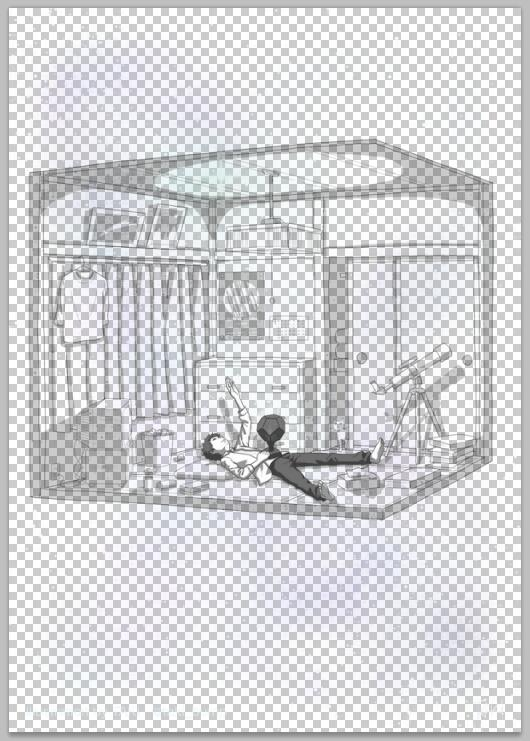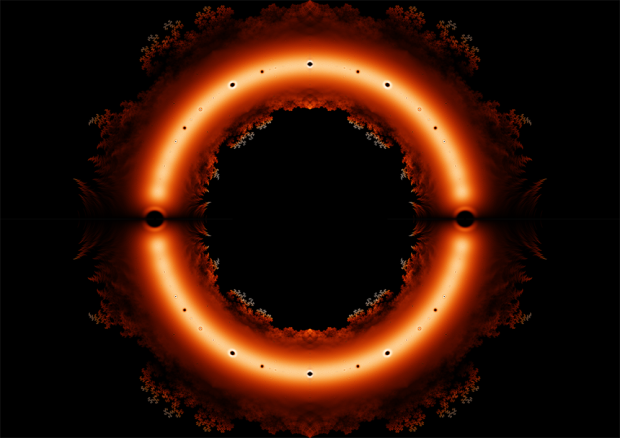## 用Python生成不同背景下显示内容不同的图片## 绘制整系数多项式复数根的分布• 如何求解多项式的根
• 图上的颜色按照什么绘制

## C++的直接初始化与复制初始化

C++中的直接初始化指的是直接调用类的构造函数进行初始化，例如

string a; //调用默认构造函数
string a("hello"); //调用参数为const char *类型的构造函数
string b(a); //调用拷贝构造函数


string a="hello";
string b=a;


## 用Mathematica拟合自定义函数

Mathematica的帮助文档是个好东西，不需要联网就可以根据你想要做的事情搜索相应的函数。

## 为什么C++直接输出21000可以精确到个位

#include<iostream>
#include<cmath>

using namespace std;

int main(){
int x;
cin>>x;
cout.precision(0);
cout<<fixed<<pow(2,x);
return 0;
}


## C/C++中的const可信吗？

C和C++中有很多函数的参数类型使用了const这个关键字。例如C语言string.h头文件中的strcpy字符串复制函数，它的原型是

char * strcpy (char * Dest, const char * Source)


void qsort (void * Base, size_t NumOfElements, size_t SizeOfElements, int (*PtFuncCompare)(const void *, const void *))


## 用freeshell提取自己百度云中一个压缩包里的文件

Linux平台上没有百度云客户端。用别人提供的Linux百度云程序不仅需要现学，而且账号的安全性不能很好的保证。于是我决定用curl命令完全模拟自己电脑上网页版百度云的下载请求。

## 用Mathematica计算物理题

1、做近似计算，略去高阶小量

$$\frac{1}{{\sqrt {{l^2} + 2lr\cos (\theta ) + {r^2}} }} + \frac{1}{{\sqrt {{l^2} – 2lr\cos (\theta ) + {r^2}} }} – \frac{2}{r}$$

Mathematica输入如下：

Assuming[r>0,Simplify[Series[-2/r+1/Sqrt[r^2+l^2-2r l Cos[\[Theta]]]+1/Sqrt[r^2+l^2+2 r l Cos[\[Theta]]],{l,0,2}]]]


$$\frac{{\left( {1 + 3\cos \left[ {2 \theta} \right]} \right){l^2}}}{{2{r^3}}} + O{\left[ l \right]^3}$$

2、在极坐标系中求梯度

Assuming[r>0,Simplify[-Grad[((1+3 Cos[2 \[Theta]]) l^2)/(2 r^3),{r,\[Theta],\[Phi]},"Spherical"]]]


$$\left\{ {\frac{{3{l^2}\left( {1 + 3\cos \left[ {2\theta } \right]} \right)}}{{2{r^4}}},\frac{{3{l^2}\sin \left[ {2\theta } \right]}}{{{r^4}}},0} \right\}$$

## 【π Day】一段很短的计算圆周率的代码

#include<stdio.h>
int a=10000,b=0,c=28000,d,e=0,f,g;
main(){
for(;b-c;)f[b++]=a/5;
for(;d=0,g=c*2;c-=14,printf("%04d",e+d/a),e=d%a)
for(b=c;d+=f[b]*a,f[b]=d%--g,d/=g--,--b;d*=b);
}